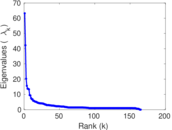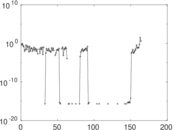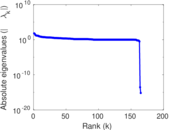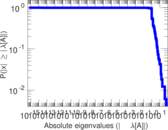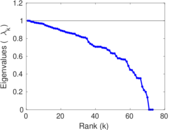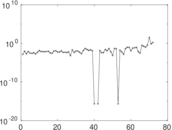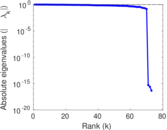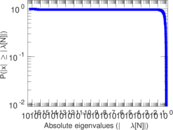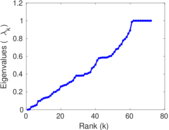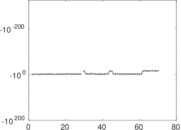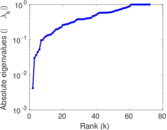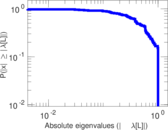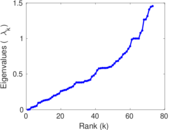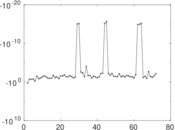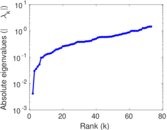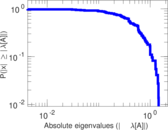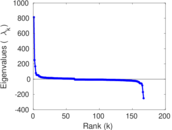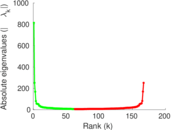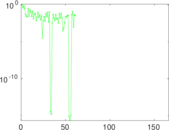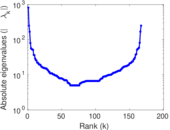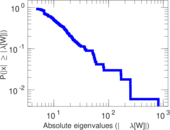# Wikibooks edits (pa)

This is the bipartite edit network of the Punjabi Wikibooks. It contains users and pages from the Punjabi Wikibooks, connected by edit events. Each edge represents an edit. The dataset includes the timestamp of each edit.

 Code `bpa` Internal name `edit-pawikibooks` Name Wikibooks edits (pa) Data source http://dumps.wikimedia.org/ AvailabilityDataset is available for download Consistency checkDataset passed all tests Category Authorship network Dataset timestamp 2017-10-20 Node meaning User, article Edge meaning Edit Network formatBipartite, undirected Edge typeUnweighted, multiple edges Temporal dataEdges are annotated with timestamps

## Statistics

 Size n = 824 Left size n1 = 167 Right size n2 = 657 Volume m = 1,278 Unique edge count m̿ = 810 Wedge count s = 22,544 Claw count z = 1,068,135 Cross count x = 45,209,071 Square count q = 269 4-Tour count T4 = 94,216 Maximum degree dmax = 212 Maximum left degree d1max = 212 Maximum right degree d2max = 75 Average degree d = 3.101 94 Average left degree d1 = 7.652 69 Average right degree d2 = 1.945 21 Fill p = 0.007 382 50 Average edge multiplicity m̃ = 1.577 78 Size of LCC N = 574 Diameter δ = 13 50-Percentile effective diameter δ0.5 = 5.255 99 90-Percentile effective diameter δ0.9 = 7.925 61 Median distance δM = 6 Mean distance δm = 5.582 95 Gini coefficient G = 0.641 319 Balanced inequality ratio P = 0.249 609 Left balanced inequality ratio P1 = 0.191 706 Right balanced inequality ratio P2 = 0.336 463 Relative edge distribution entropy Her = 0.862 098 Power law exponent γ = 5.053 97 Tail power law exponent γt = 2.631 00 Tail power law exponent with p γ3 = 2.631 00 p-value p = 0.002 000 00 Left tail power law exponent with p γ3,1 = 1.881 00 Left p-value p1 = 0.238 000 Right tail power law exponent with p γ3,2 = 4.141 00 Right p-value p2 = 0.249 000 Degree assortativity ρ = −0.164 707 Degree assortativity p-value pρ = 2.445 46 × 10−6 Spectral norm α = 63.201 0 Algebraic connectivity a = 0.004 174 71 Spectral separation |λ1[A] / λ2[A]| = 1.496 33 Controllability C = 494 Relative controllability Cr = 0.602 439

## Plots

### Fruchterman–Reingold graph drawing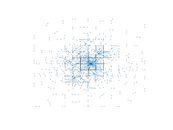### Degree distribution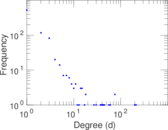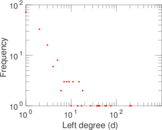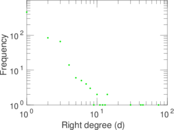### Cumulative degree distribution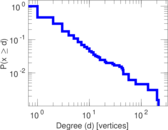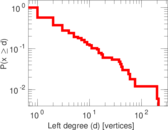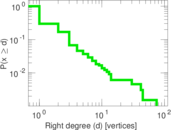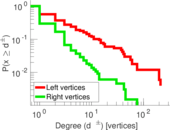### Lorenz curve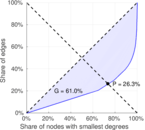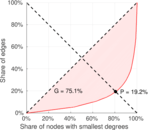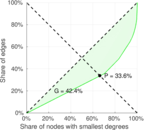### Spectral distribution of the adjacency matrix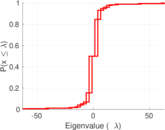### Spectral distribution of the normalized adjacency matrix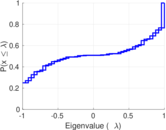### Spectral distribution of the Laplacian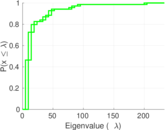### Spectral graph drawing based on the adjacency matrix### Spectral graph drawing based on the Laplacian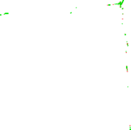### Spectral graph drawing based on the normalized adjacency matrix### Degree assortativity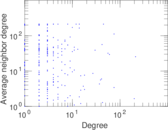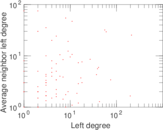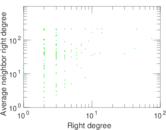### Zipf plot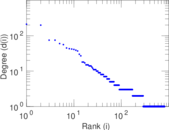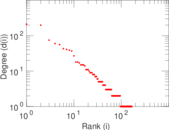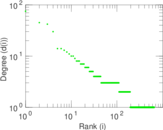### Hop distribution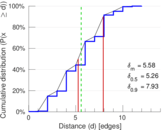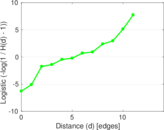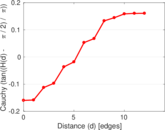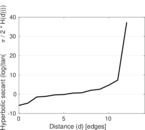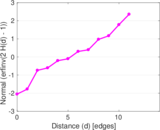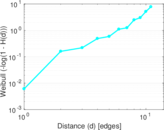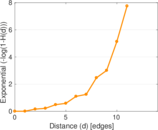### Double Laplacian graph drawing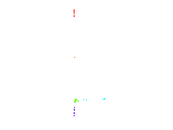### Delaunay graph drawing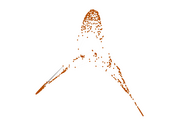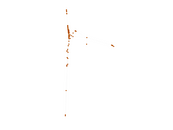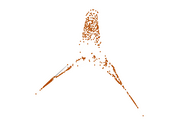### Edge weight/multiplicity distribution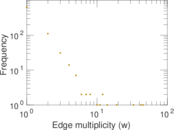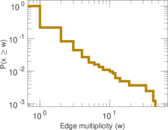### Temporal distribution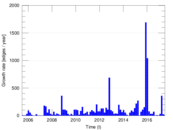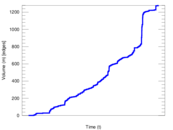### Temporal hop distribution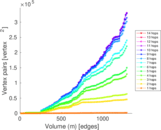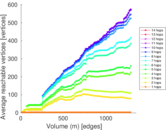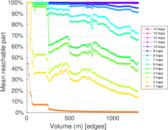### Diameter/density evolution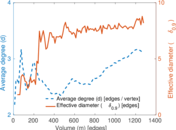### Matrix decompositions plots